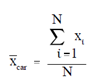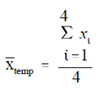## Average Value Assignment Help

Assignment Help: >> Averages - Average Value

Average Value:

The summation symbol, Σ, introduced in the first chapter, is often used when dealing with the average value, x¯ .

Using the first example here, the average value could have been expressed in the following manner:where:

car        =          the average value (cost) of a car

xi         =          each of the individual car prices

N         =          total number of cars

In the right side of the above equation can then be rewritten.

car  = x1 + x2 + x3 + x4 + x5 + x6/6

Substituting the value 10,200 for x1, 11300 for x2, 9,900 for x3, etc.

car  =     10,200 + 11,300+  9,900+  12,000+  18,000+  7600/6

car  = 11,500

Example:

If we were to apply the average value equation from above to the second example concerning temperature so how would it be written and what would be the values for N1, xi?

Solution:x1  = 600

x2  = 596

x3  = 597

x4  = 603

temp   = x1 + x2 + x3 + x4/4

(600 + 596 +597 + 603)/4

=          599